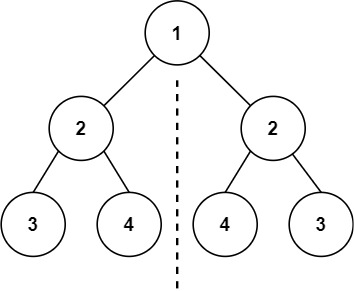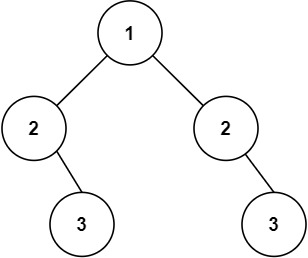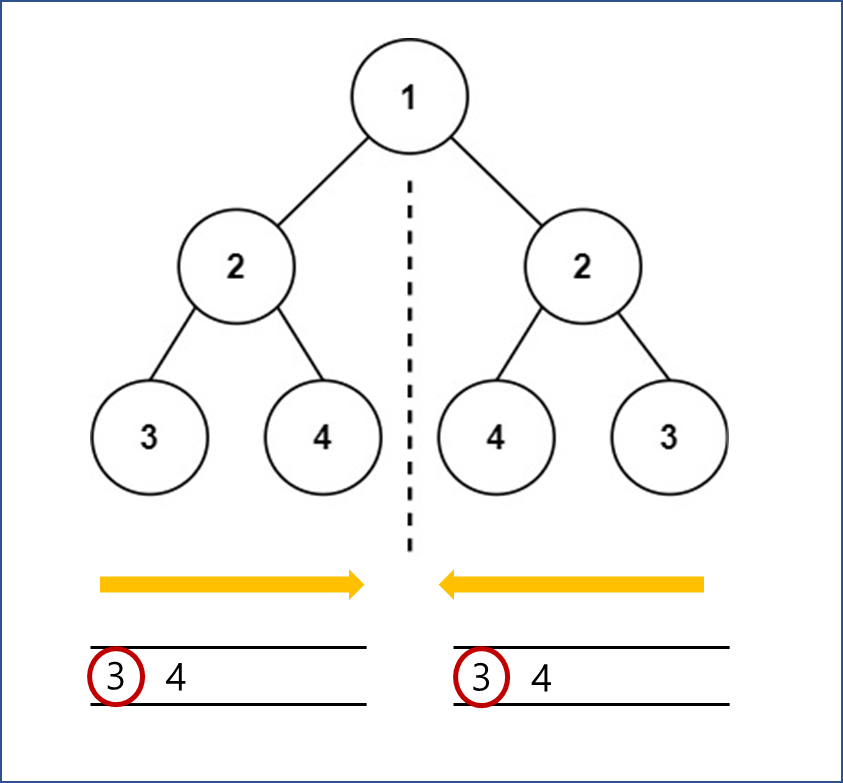# [Leetcode]101. Symmetric Tree

limelimejiwon·2022년 3월 22일
0

## Python으로 공부하는 코딩테스트

목록 보기
20/67## 📄 Description

Given the root of a binary tree, check whether it is a mirror of itself (i.e., symmetric around its center).

### Example 1:Input: root = [1,2,2,3,4,4,3]
Output: true

### Example 2:Input: root = [1,2,2,null,3,null,3]
Output: false

## 🔨 My Solution

• use Stack with iterative approach

🔴 Variables
left : nodes of the left parts
right : nodes of the right parts

### 🔵 Rules

1) Conditions for Symmetric Tree
- If the both left and right node is None, its Symmetric.
- If the one of the left and right node is None, its not Symmetric.
- If the value is different, its not symmetirc. In other words, the value have to be same to be a symmetric tree.

2) How to check the child nodes : BFS Search
- pop the left element(pop(0)) from the left and right.
- For the left node's child, append the left.left first and then left.right.
- For the right node's child, append the right.right first and then right.left.

### Why is the order you add to queue different?If you see the above picture, you will understand why.
For the child node(both left and right) popped off from the left should be put in order of left->right, and the child node(both left and right) popped off form the right should be put in order of right->left.

## 💻 My Submission

class Solution:
def isSymmetric(self, root: Optional[TreeNode]) -> bool:
'''
left: left part nodes
right: right part nodes
'''

left=[root.left]
right=[root.right]

while left and right:
l_node=left.pop(0)
r_node=right.pop(0)
# if the both l_node and r_node is None, its symmetric
if not l_node and not r_node:
continue
# if one of the two is None, its not symmetric
if not l_node or not r_node:
return False
# if the value is different, its not symmetirc
if l_node.val!=r_node.val:
return False
# append the left child and right node
left.append(l_node.left)
left.append(l_node.right)
right.append(r_node.right)
right.append(r_node.left)
return True

Could you solve it both recursively and iteratively?

## Other Solution (1) Recursion

class Solution:
def isSymmetric(self, root: Optional[TreeNode]) -> bool:

return self.recursion(root.left, root.right)

def recursion(self, l_node,r_node):
if l_node is None and r_node is None:
return True
if l_node is None or r_node is None:
return False

if l_node.val==r_node.val:
return self.recursion(l_node.left, r_node.right) and self.recursion(l_node.right, r_node.left)
else:
return False